# IQ Test Labs

touch_app

### Definition

In mathematics a number sequence is an ordered list of objects. The members of the list are also called elements or terms.

The length of a sequence can be finite or infinite. It may be infinite such as the sequence of all positive odd integers {1, 3, 5, 7, ...}, or finite such as ten to five backwards {10, 9, 8, 7, 6, 5}.

In sequences the order matters. Repetition is allowed and a term can appear in multiple locations {3, 3, 6, 6, 9, 12, 12, 24, ...}

### Sequence formulas

Standard sequence notation: {a1, a2, a3, a4, ...}

• an = nthelement
• n = term position
• a1 = first term

In formulas, 'd' denotes the common difference between the terms (arithmetic progressions), and 'r' denotes the common ratio between the terms (geometric progressions).

In order to compute the nth term of a sequence it is necessary to have a general formula.

Example:

If the general formula for a sequence is an = 3n + 2, then the 3rd term would be:

a3 = 3(3) + 2 = 11

A recurrence relation is an equation that defines a sequence where each term is a function of the preceeding term.

Example:

The sequence of square numbers.

{1, 4, 9, 16, 25, 36, 49, 64, 81, ...}

The recurrence relation is: an = an-1 + 2n-1

a4 = a3 + 2(4)-1

a4 = 9 + 7 = 16

### Question structure

• Sequences usually consist of 4-7 terms and one or two missing numbers.
• When there is one missing number, it is usually toward the end, but can also be at the beginning or middle.
• More missing numbers may add a layer of difficulty since less terms are available for discovering a pattern or formula.
• Usually the rule is inferred from the first few items, and a sequence may appear more difficult when the missing item appears early.

Questions commonly ask for missing number(s). They may also ask to derive a formula or to find which term is the odd one out.

### Checklist

• Use addition or subtraction to check for a common difference and a possible arithmetic progression.
• Check for a geometric progression by multiplying or dividing the terms to find a common ratio.
• Do the terms in the sequence follow a repeating pattern?
• Does the sequence consist of repeatedly squaring the terms?
• Check for a triangular sequence. Does each term create a larger triangle?
• Are all items raised to the power of three? If so the series is a cube series.
• Does each term form the basis of a larger pentagonal shape?
• Does each term describe the sides of a larger hexagonal shape?
• Do the position of the terms in the sequence create significance?
• If the terms reach a certain point and revert to the initial value, the sequence is cyclic.
• A series may be the same or similar to a Fibonacci series if items are sums of preceeding terms.
• If the series is erratic then it may be a twin sequence, where two independent sequences are combined into one larger sequence.
• Are multiple operations applied to each term or specific terms?
• Check for odd/even number progressions
• Check for prime/composite numbers sequences.
• Are there any noticeable patterns that apply to number digits?
• Check to see if terms are multiples of a number.
• Do you notice patterns that occur in the differences between consecutive terms? Differences between consecutive terms are commonly multiples, perfect cubes, arithmetic progressions, or geometric progressions.
• Can you discern patterns that occur in the ratios between consecutive terms? Ratios between consecutive terms are commonly prime numbers, arithmetic/geometric progressions, perfect squares/cubes, and multiples.
• If the rate of increase is sharp at first and subsequently levels off, the terms of the sequence may contain exponents.
• If terms are getting bigger and the rate of increase isn't leveling off, the sequence may contain factorials.
• It's important to keep an open mind, and be creative; sequences follow all sorts of rules and the possibilities are endless. Figuring out the the lazy caterer’s sequence is one such example.

### Sequence types

#### Arithmetic progression

• The same number is added or subtracted to each preceeding term.
• The difference, 'd', between consecutive terms may be positive or negative, and is always constant.
• We can check for an arithmetic progression by using addition of subtraction to find a common difference.

Common difference: Successive term – Preceding term

Example 1

Common difference is positive:

{5, 10, 15, 20, 25, 30, 35, 40, 45, 50, ...}

d = 5

Example 2

Common difference is negative:

{25, 23, 21, 19, 17, 15, ...}

d = -2

The formula for finding the nth term, without making reference to, and listing the previous terms is:

an = a1 + (n-1)d

where 'a1' is the first term and 'd', is the common difference.

Recurrence relation: an = an-1 + d

#### Geometric progression

In a geometric progression each term is multiplied by a common ratio 'r'.

The common ratio can be calculated by dividing any two consecutive terms.

Check for a geometric relationship by multiplying successive terms to see if the ratio is constant.

Common ratio: Successive term/Preceding term

Example 1

Increasing geometric sequence.

{2, 4, 8, 16, 32, 64, 128, 256, 512, ...}

r = 2

Example 2

Decreasing geometric sequence.

{2187, 729, 243, 81, 27, 9, 3, ...)

r = 1/3

Example 3

The common ratio may be less than one. For example if it's a half, the sequence will look like this:

{10, 5, 2.5, 1.25, 0.625, 0.3125, ...}

r = 0.5

Example 4

The common ratio may be negative. In this case, the sequence will alternate between positive and negative:

{1, -4, 16, -64, 0.625, -256, ...}

r = -4

Example 5

In music the note A can be played in the following frequencies:

{110Hz, 220Hz, 440Hz, 880Hz, ...}

r = 2

Each frequency is multiplied by the same number each time.

Each frequency is a pitch, and the difference between pitches is an octave.

General term (nth term)

an = a1r(n-1)

where a1 is the first term and r is the common ratio.

Recurrence relation: an = (an-1)r

Attempt more multiplication/division questions.

#### Patterned

The terms in the sequence follow a repeating pattern. The numbers are usually increasing, decreasing, constant or alternating.

Example 1

{1, 0, 1, 1, 0, 1, ?, 1, 0, 1, 1, 1, 1, 0, 1, 1, 1, 1, ?, 0}

Explanation: There is an increasing order of 'ones' between the zeros.

Example 2

{1, 33, 666, ?, 1212121212, 151515151515, ?, 2121212121212121}

Explanation: There are one 1s, two 3s, three 6s, four 9s etc

Attempt more patterns questions.

#### Sequence of square numbers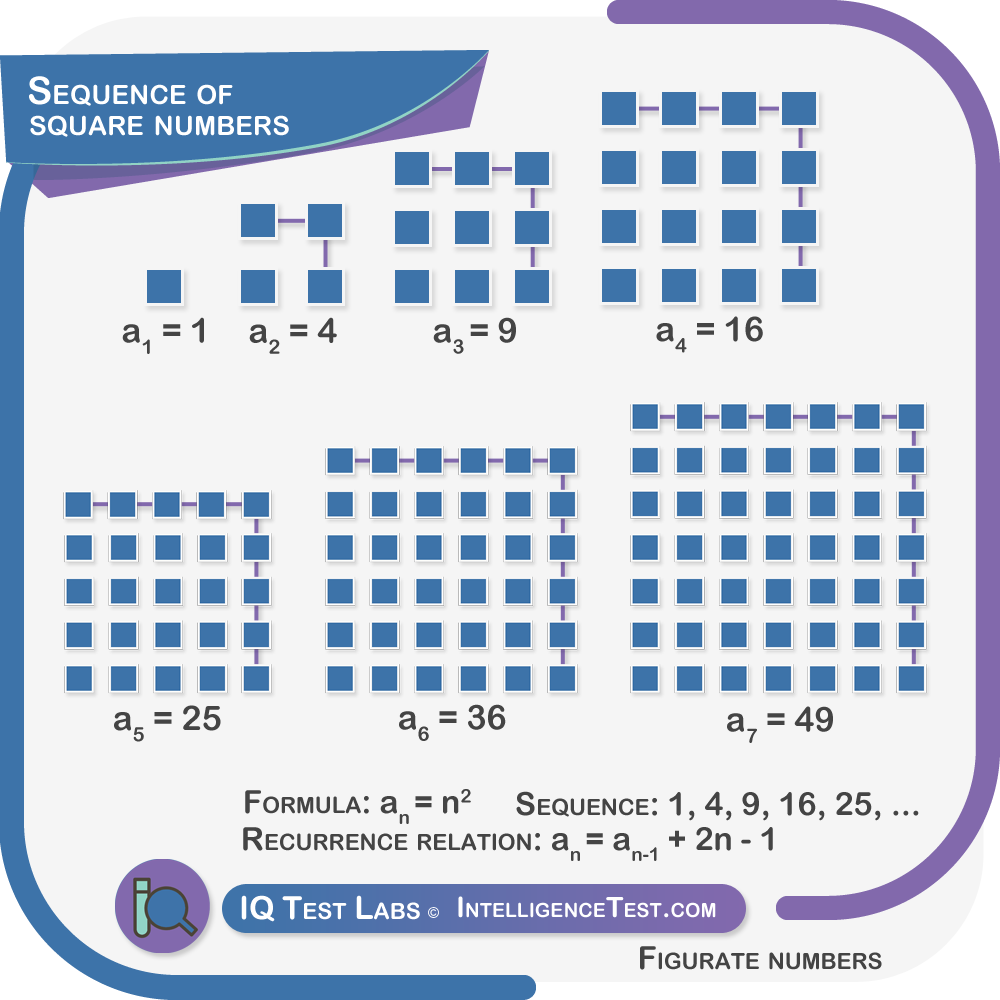• Type of figurate number.
• Perfect squares are the squares of whole numbers.
• Square numbers are always positive.

Example:

{1, 4, 9, 16, 25, 36, 49, 64, 81, ...}

This sequence consists of repeatedly squaring numbers {1, 2, 3, 4, ...}

Formula: an = n2

Recurrence relation: an = an-1 + 2n-1

Attempt figurate number questions.

#### Sequence of triangular numbers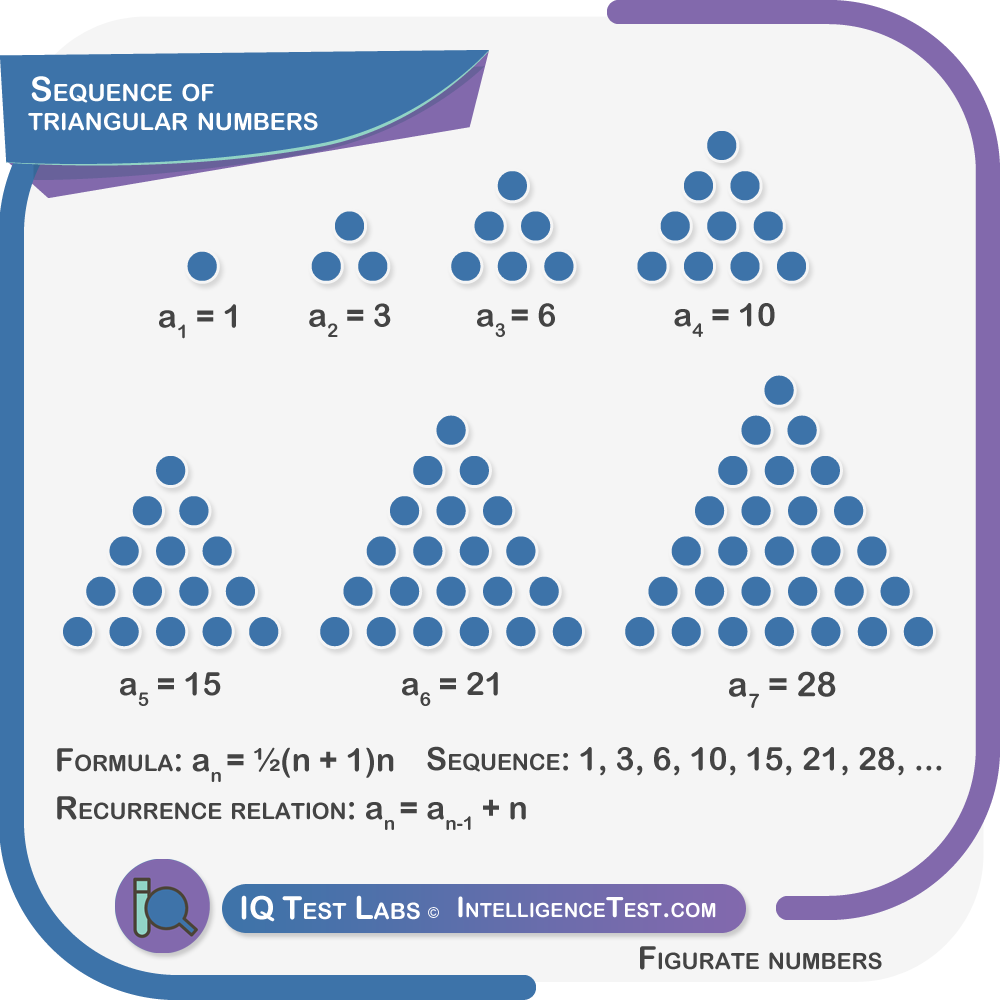• Type of figurate number.
• The terms in the sequence represent the number of dots needed to form successive equilateral triangles.
• A new term is added to the sequence after each new triangle row.

In the triangular number sequence, each term represents a new row of dots.

1

1+2=3

1+2=3=6

1+2+3+4=10

1+2+3+4+5=15

1+2+3+4+5+6=21

1+2+3+4+5+6+7=28

Formula: an = ½(n + 1)n

Recurrence relation: an = an-1 + n

Attempt figurate number questions.

#### Cube series

A cube is obtained by multiplying a number by itself three times.

Formula: an= n3

Example

{1, 8, 27, 64, 125, 216, 343, 512, 729, ...}

1 = (1×1×1), 8 = (2×2×2) 27 = (3×3×3) 64 = (4×4×4) ...

What is the next number in the sequence?

Since it's the 10th number, the answer is 1,000.

1010, or 10*10*10= 1,000.

Attempt more figurate number questions.

#### Pentagonal number sequence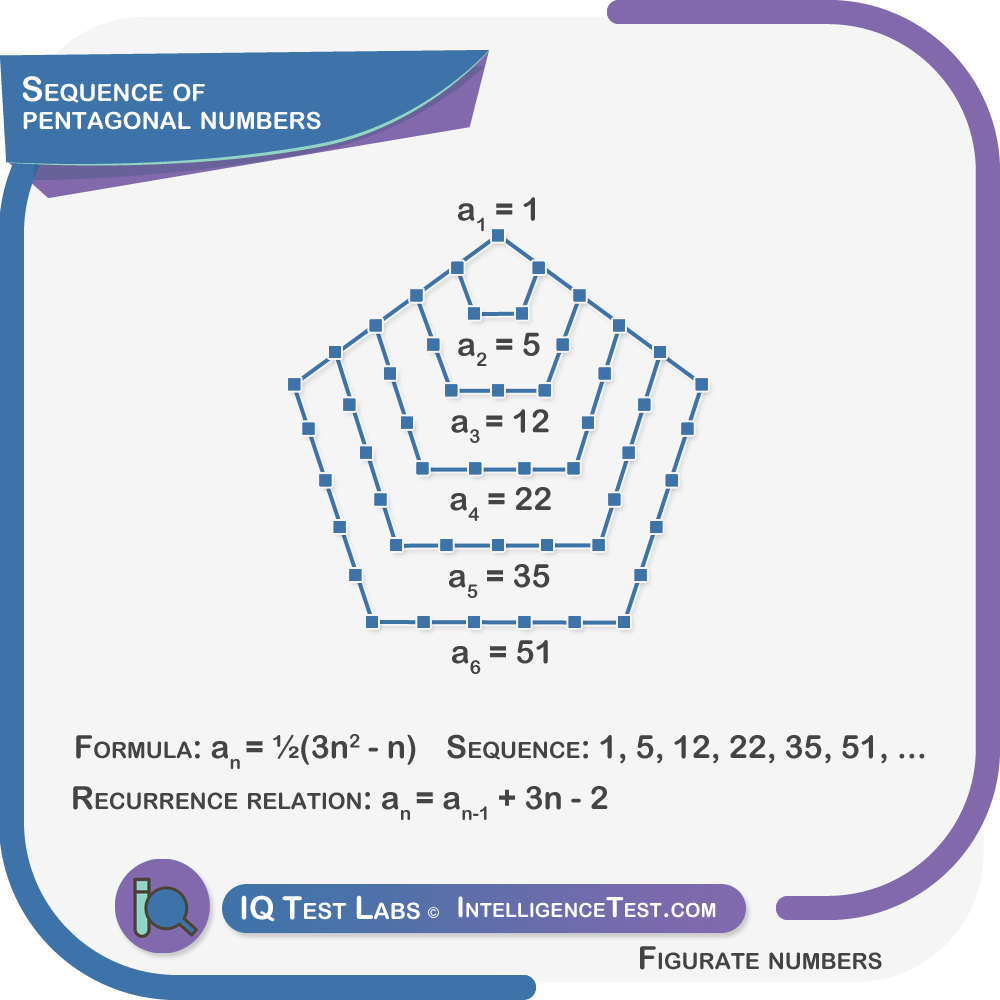• Type of figurate number.
• The first pentagonal numbers are 1,5,12,22,35,51,70,92, ...
• This sequence consists of the patterns of dots that outline regular pentagons.
• The pentagons share a common vertex.
• Pentagonal numbers are the number of distinct dots that outline each shape.

Formula: an = ½(3n2 - n)

Recurrence relation: an = an-1 + 3n - 2

Attempt figurate number questions.

#### Hexagonal number sequence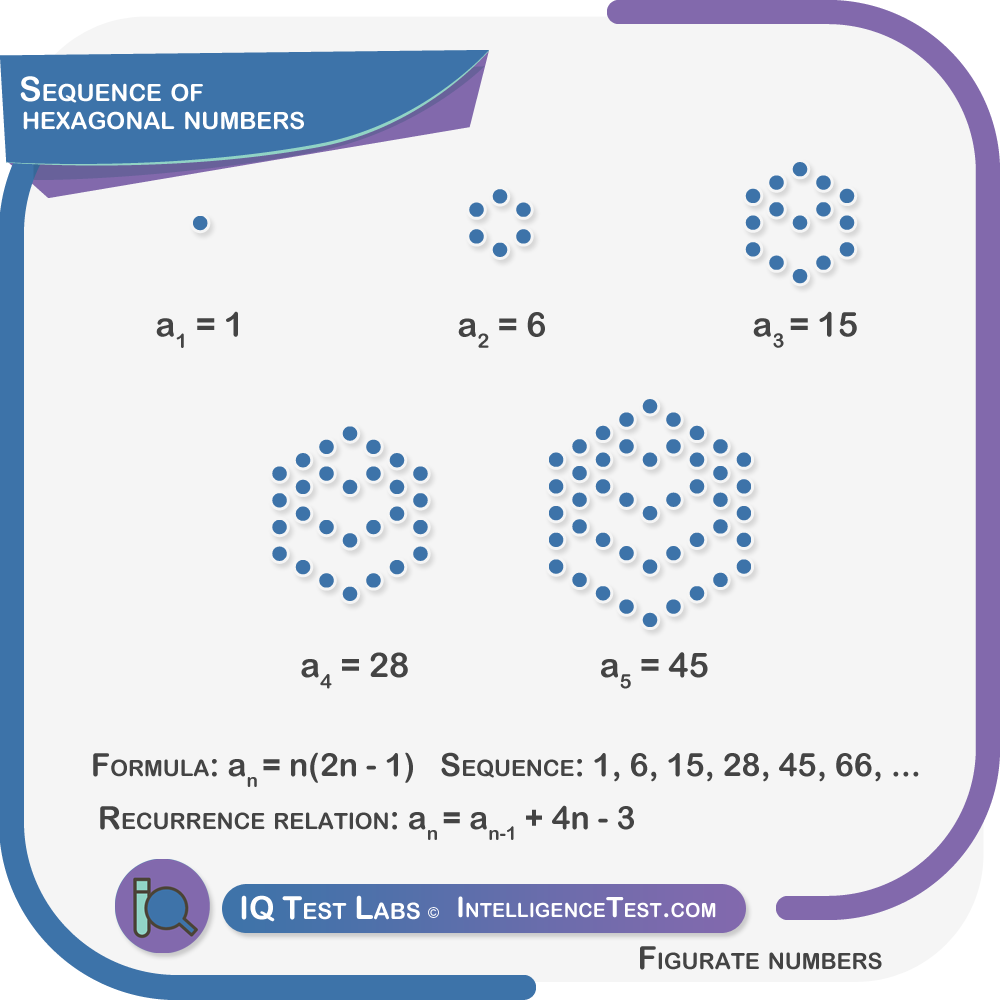• Type of figurate number.
• The first hexagonal numbers are 1,6,15,28,45,66,91,120 ...
• This sequence consists of the patterns of dots that outline regular hexagons.
• The hexagons share a common vertex.
• Hexagonal numbers are the number of distinct dots that outline each shape.

Formula: an = n(2n − 1)

Recurrence relation: an = an-1 + 4n - 3

Example

What is the sixth hexagonal number?

Replacing n with 6

a6 = 6(2 × 6 − 1) = 6(12 − 1) = 6 × 11 = 66

Attempt more figurate number questions.

#### Positions of terms

The position of the terms in a sequence may facilitate formulating a rule.

For example, this sequence may not, at first glance, be identified as a series of cubes.

{1,8,27,64,125, ?}

After an initial review we notice

• no multiplication/division pattern.
• no pattern in the term differences (+7,+19,+37,+61)

The cube series becomes more easily apparent after associating terms with their sequence positions.

{1, 8, 27, 64, ...}

|   |   |   |   |

1  2  3  4  5

1^3=1, 2^3=8, 3^3=27, 4^3=64, 5^3=125

#### Cyclic sequences

Some sequences demonstrate cyclic patterns; they reach certain values and then revert to their initial value.

An appropriate illustration is a time value sequence. Time increments coincide with a return to the initial time.

Example 1

Common difference is positive:

{16:45, 16:30, 16:15, 16:00, 15:45, 15:30, 15:15, ?}

Explanation: Subtract 15 minutes from the preceeding term.

Example 2

{2123, 1232, 2321, 3212, 2123, 1232, 2321, 3212, ?, ?, ?, ?}

Explanation: A four number cycle where the first digit is moved back. The terms repeat every four cycles.

Attempt more cyclic pattern questions.

#### Fibonacci series

By definition, the first two numbers are 0, and 1 (a0 = 0, a1 = 1)

All terms are obtained by adding two previous terms.

Terms in a Fibonacci series can be obtained with the formula:

an = an-1 + an-2

a6 = a6-1 + a6-2 = a5 + a4 = 5 + 3 = 8

{0, 1, 1, 2, 3, 5, 8, 13, 21, 34, 55, 89, 144, 233, 377, 610, ...}

#### Twin sequences

A combination of two series into a single series. It is therefore necessary to analyze each sequence separately.

Example

{2, 10, 4, 20, 6, 40, 8, 80, 10, 160, 12, 320, 14, ...}

The first series is an arithmetic progression with a common difference of two:

{2, 4, 6, 8, 10, 12, 14, ...}

The second series is an geometric progression with a common ratio of two:

{10, 20, 40, 80, 160, 320, ...}

Attempt more twin sequences questions.

#### Multiple operations

Multiple operations are applied to terms in a sequence, or separate operations are applied to separate terms.

Example 1

{4, 14, 44, 134, 404, 1214, ?, 10,934, ...}

Explanation: Multiply each term by three and add two.

Example 2

{5,10,8,13,?,16,14, 19, 17, 22, ?}

Explanation: Add five to the first term and subtract two from the next term.

Attempt more multiple operations questions.

#### Odd numbers

An infinite sequence of odd numbers.

{1, 3, 5, 7, 9, 11, 13, 51, 17, 19, 21, ...}

#### Even numbers

An infinite sequence of even numbers.

{2, 4, 6, 8, 10, 12, 14, 16, 18, 20, ...}

#### Prime numbers

A number that is divisible only by one and itself.

{2, 3, 5, 7, 11, 13, 17, 19, 23, 29, 31, ...}

#### Composite numbers

Composite numbers are whole numbers that have two or more factors.

Whole numbers that are not prime numbers are composite numbers.

{4, 6, 8, 9, 10, 12, 14, 15, 16, ...}

#### Digits

• Distinct patterns in the digits of terms.
• In a two-digit number for example, the first digit might increase, while the second digit remains constant.
• Digits could follow a cycle or add up to a specific number.

Example 1

{100, 219, 328, 437, 546, 655, 764, ?}

Explanation: The first two digits increase by one, and the third digit decreases by one.

Example 2

{12, 34, 56, 78, 10, 32, 54, 76, 18, 30, ?, ?}

Explanation: The first digits alternate between 1,3,5,7, and the second digits alternate between 2,4,6,8,0.

Attempt more digits sequence questions.

#### Multiples of numbers

Multiples of a number are those that will divide into the number exactly.

Example:

Multiples of six

{6, 12, 18, 24, 30, 36, ...}

#### Term differences

Patterns that occur in the differences between consecutive terms.

Multiples

{2, 6, 14, 30, 62, ...}

The differences between the terms are multiples of four:

4, 8, 16, 32.

Perfect cubes

{6, 14, 41, 105, ...}

The differences between the terms are all cubes.

23, 33, 43.

Arithmetic progression

{2, 3, 6, 11, 18, ...}

The differences between the terms have a common difference

1, 3, 5, 7

Geometric progression

{2, 3, 6, 15, 42, ...}

The differences between the terms have a common ratio.

1, 3, 9, 27

Attempt more term-difference questions.

#### Term ratios

Patterns that occur in the ratios between consecutive terms.

Constant ratio

{2, 8, 32, 128, 256, ...}

The ratio between each term is always four.

4, 4, 4, 4

The next term is 4 x 256 = 512

Prime numbers

{11550, 1050, 150, 30, 10, ...}

The ratio between each term is a decreasing prime.

11, 7, 5, 3

The next term is 10÷2 = 5

Arithmetic progression

{1, 4, 24, 192, 1920, ...}

The ratio between each term is in an arithmetic progression.

4, 6, 8, 10,

The next term is 12 x 1920 = 23040

Perfect square

{1, 4, 36, 576, ...}

The ratio between each term is a perfect square

4(22) 9(32) 16(42)

The next term is 25 x 576 = 14400

Multiples

{1, 3, 18, 162, ...}

The ratio between each term is multiples of 3

3, 6, 9

The next term is 12 x 162 = 1944

Perfect cube

{1, 8, 216, 10,368, ...}

The ratio between each term is a perfect cube

8(23) 27(33) 64(43)

The next term is 125 x 10368 = 1.296M

Geometric progression

{1 1 3 27 729, ...}

The ratio between each term is in geometric progression.

1, 3, 9, 27

The next term is 81 x 729 = 59049

#### Exponents

An exponent refers to the times a number is multiplied by itself.

In number sequences, numbers are generally raised to the power of two (a perfect square) or to the power of three (a perfect cube).

Example 1

{1, 3, 9, 27, 81, ?}

Explanation: Three to the power of zero through five.

30=1, 31=3, 32=9, 33=27, 34=81, 35=243

Example 2

{1, ?, 625, 15625, 380625}

Explanation: Five to the power of all even numbers from zero to eight.

50=1, 52=25, 54=625, 56=15,625, 58=380,625

Attempt more exponents questions.

#### Factorials

Factorials involve multiplying an integer with all the integers below it. For example factorial three, or 3! is 3x2x1 = 6.

Addition, division, and squaring are prominient for factorials that feature in number sequences.

Example

{1, 2, 3, 6, 24, ?, 720, 5040}

5! = 5 x 4 x 3 x 2 x 1 = 120.

Explanation: Series of factorials, zero through seven. Remember that 0! = 1.

#### Creative solutions

A sequence can be made up of any list of items, and this is what makes some sequences more complex than they actually are.

When a sequence is erratic, it's usually a simple rule that has to be discovered via trial and error. Keep an open mind and try alternative patterns until something makes sense.

Example 1

This sequence is all even numbers without a one in them.

{2, 4, 6, 8, 20, 22, ...}

Example 2

This sequence is square odd numbers that contain the number nine.

{9, 49, 169, 289, ...}

Example 3

Numbers may be in alphabetical order

{8, 5, 4, 9, 1, 7, 6, 10 ...}

Example 4

Successive digits of

{3, 1, 4, 1, 5, 9, 2, 6 ...}

Example 5

For each third term, multiply the two preceeding terms.

9*5 = 45, 8*6 - 48, ...

{9, 5, 45, 8, 6, 48, 2, 6, 12, ...}

#### The Lazy Caterer’s Sequence

The lazy caterer’s sequence describes the maximum number of possible pieces that a disk can be divided into, with a given number of straight cuts.

The maximum circle segments that can be made with a given number of straight cuts.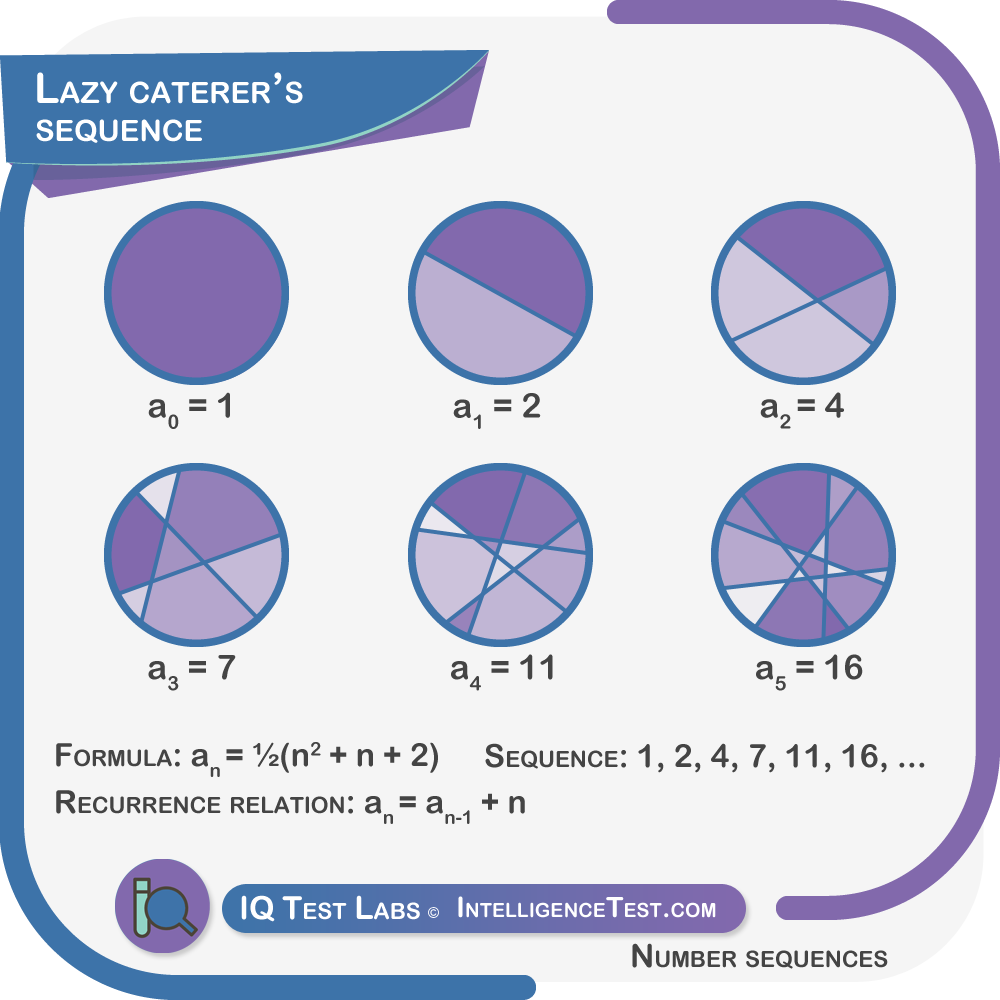Formula: an = ½(n2 + n + 2)

Recurrence relation: an = an-1 + n

{1, 2, 4, 7, 11, 16, 22, 29, 37, ...}

#### Look and say sequence

This sequence results by reading and saying the digits of a sequence.

Example:

{1, 11, 21, 1211, 111221, 312211, 13112221, 1113213211, ...}

It's as if there were an inventory check of numbers.

The reader would say "there is one, 1"

This makes the next term '1,1'.

The next term would be read out loud as, "two 1s" i.e '21'.

The term after that would be "one 2", i.e '12', followed by "one 1", i.e, '11', resulting in '1211'.

### General formulas

These are tips and general guidelines for defining sequence formulas.

Case 1:

Check for addition or subtraction differences between the terms of the sequence.

Example:

{4, 7, 10, 13, ...}

In this case, the differences are, 3,3,3 which is regular.

n | term

1 |   4

2 |   7

3 |   10

4 |   13

A regular pattern could point to a rule where multiplication plays a role.

The regular difference of three, therefore, may suggest a x3 multiplication.

A new column shows 3n for each term:

n | 3n | term

1 |    3   |   4

2 |    6   |   7

3 |    9   |   10

4 |   12  |   13

Each term is 1 plus 3n, therefore the rule is an = 3n + 1

Note: If the sequence has a common difference of three, there will probably be a three in the formula.

Case 2:

Example:

{2, 5, 10, 17, 26, 37, ...}

The differences betwen the terms are: 3,5,7,9, ...

There is a regular +2 increase.

When differences are increasing regularly check for square numbers.

n | term

1 |   2

2 |   5

3 |   10

4 |   17

5 |   26

6 |   37

n |   n2   | term

1 |      1     |   2

2 |      4     |   5

3 |      9     |   10

4 |     16    |   17

5 |     25    |   26

6 |     36    |   37

Here the relationship between n2 and the term results in the formula Tn = n2 + 1

Case 3:

Example:

{3, 12, 27 , 48, 75, 108, ...}

The differences between the terms are: 9,15,21,27, and 33.

There is a regular +6 increase.

This is another regular increase, which like before, indicates square numbers:

n | term

1 |   3

2 |   12

3 |   27

4 |   48

5 |   75

6 |   108

n |   n2   | term

1 |      1     |   2

2 |      4     |   12

3 |      9     |   27

4 |     16    |   48

5 |     25    |   75

6 |     36    |   108

Here the relationship between n2 and the terms results in the formula an = 3n2

Case 4:

Example:

{2, 6, 12, 20, ...}

The differences are, 4,6,8, another regular increase, which again indicates square numbers:

n | term

1 |   2

2 |   6

3 |   12

4 |   20

n |   n2   | term

1 |      1     |   2

2 |      4     |   6

3 |      9     |   12

4 |     16    |   20

5 |     25    |   30

Here the relationship between n2 and the term results in the formula an = n2+n

Finding the rule is guesswork more than anything else, and it mostly comes down to remembering past techniques, and trying random new ones.